Engineering Mechanics

# Engineering Mechanics

Test Description

## 15 Questions MCQ Test GATE Mechanical (ME) 2023 Mock Test Series | Engineering Mechanics

Engineering Mechanics for Mechanical Engineering 2023 is part of GATE Mechanical (ME) 2023 Mock Test Series preparation. The Engineering Mechanics questions and answers have been prepared according to the Mechanical Engineering exam syllabus.The Engineering Mechanics MCQs are made for Mechanical Engineering 2023 Exam. Find important definitions, questions, notes, meanings, examples, exercises, MCQs and online tests for Engineering Mechanics below.
Solutions of Engineering Mechanics questions in English are available as part of our GATE Mechanical (ME) 2023 Mock Test Series for Mechanical Engineering & Engineering Mechanics solutions in Hindi for GATE Mechanical (ME) 2023 Mock Test Series course. Download more important topics, notes, lectures and mock test series for Mechanical Engineering Exam by signing up for free. Attempt Engineering Mechanics | 15 questions in 45 minutes | Mock test for Mechanical Engineering preparation | Free important questions MCQ to study GATE Mechanical (ME) 2023 Mock Test Series for Mechanical Engineering Exam | Download free PDF with solutions
 1 Crore+ students have signed up on EduRev. Have you?
Engineering Mechanics - Question 1

### Directions: Q. 1 - 5  carry 1 mark each A constant force of 10 N is acting on a body of mass ‘m’ for 10 seconds and changes its velocity from 10m/s to 15m/s. Calculate the mass of the body

Detailed Solution for Engineering Mechanics - Question 1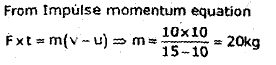Engineering Mechanics - Question 2

### A solid cylinder of diameter 5.0 m has a height of 6.0 m. Find the meta-centric height of the cylinder if the specific gravity of the material of cylinder 0.45 and it is floating in water with its axis vertical. State whether the equilibrium is stable or unstable.

Detailed Solution for Engineering Mechanics - Question 2

BG=Centre of pontoon – Centre of immersed portion=0.3-0.45*0.3=1.65
Metacentric height=I/∀ -BG
I=π*r⁴=π*2.5⁴
∀=π*r*r*h=π*2.5*2.5*6
Metacentric height=-0.61.

Engineering Mechanics - Question 3

### The ratio of limiting friction and normal reaction is known as

Engineering Mechanics - Question 4

If Fx and Fy are algebraic sum of horizontal forces and vertical forces at a point under equilibrium condition, then angle made by resultant force with horizontal direction is

Engineering Mechanics - Question 5

If three forces acting in one plane upon a rigid body, keeping it in equilibrium, then they must either

Engineering Mechanics - Question 6

A toggle joint with equal arms is shown in figure. Find out the resistance offered by joint ‘A’ on horizontal direction.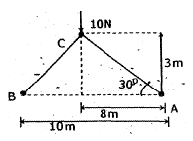Detailed Solution for Engineering Mechanics - Question 6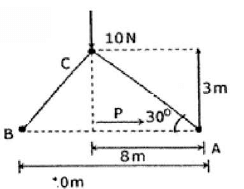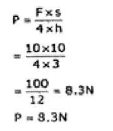Engineering Mechanics - Question 7

What is the ratio of radius of gyrations of two bars of small diameters, in first case axis is at end and in other case axis at centre of bar?

Detailed Solution for Engineering Mechanics - Question 7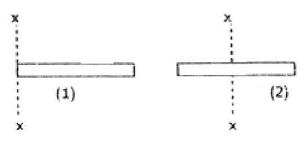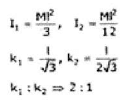Engineering Mechanics - Question 8

A 50 kg block is pulled up on 30° inclined plane by a constant force 800 N. Co-efficient of friction between block and plane is 0.5. What is the velocity of block after 10 seconds?

Detailed Solution for Engineering Mechanics - Question 8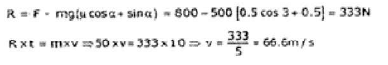Engineering Mechanics - Question 9

For a hexagonal cross sectional bar as per designer specification held in such a way that the total bar is having line contact with ground, but manufacturer placed that bar in such a way that it has area contact with ground. In which case it has more strength?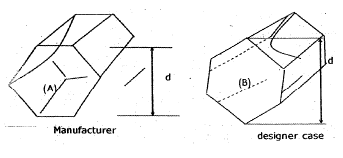Detailed Solution for Engineering Mechanics - Question 9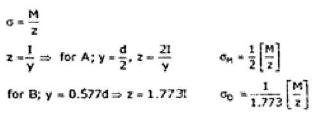Engineering Mechanics - Question 10

A differential pulley is used to lift a weight of 200 N as shown in figure. What is the minimum force required to lift the weight? Take smaller pulley radius as 2 m and larger pulley radius as 5 m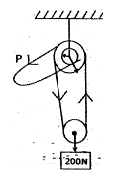Detailed Solution for Engineering Mechanics - Question 10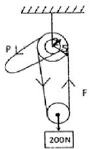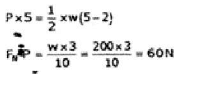*Answer can only contain numeric values
Engineering Mechanics - Question 11

For an equilateral triangle ABC, center of gravity is at the centre of circle which is inscribed in a triangle drawn at the midpoints of side of triangle. The distance of centre of gravity from side AB is _________ m.

Detailed Solution for Engineering Mechanics - Question 11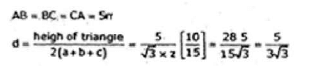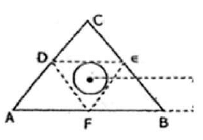Engineering Mechanics - Question 12

A solid pyramid height is 10m. Find out the centre of gravity distance from the base of pyramid.

Detailed Solution for Engineering Mechanics - Question 12

a = h/4 = 10/4 = 2.5 m

Engineering Mechanics - Question 13

A ladder rests against a frictionless wall. The coefficient of friction between ladder and the ground is 0.8. What is the smallest angle θ at which the ladder will remain stationary?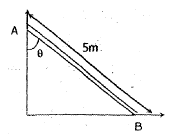Detailed Solution for Engineering Mechanics - Question 13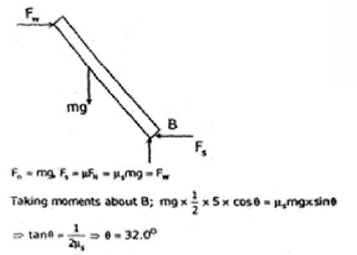Engineering Mechanics - Question 14

A person of weight 60 kg and carrying a bag weight of 20 kg. He tries to climb wall where ladder of mass 200 kg is placed, co-efficient of reaction between wall and ladder is 0.8 and ladder and ground is 0.9. When he is at 3/5 of the way up the ladder, it starts to slip. What was the angle between ladder and land when he starts climbing?

Detailed Solution for Engineering Mechanics - Question 14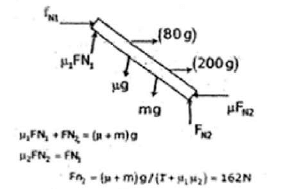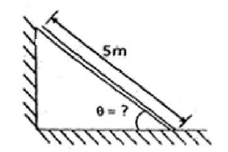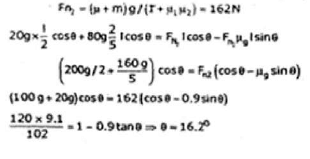Engineering Mechanics - Question 15

A car moving with 96 kmph decides to overtake a truck which is moving with 96 kmph. So car accelerates the speed up to 120 kmph and immediately starts to decelerate until its speed again dropped to 96 kmph. The whole process takes 30 seconds, if the gap between car and truck was 35 m and truck is 10 m long while car is 4m long. What will be the gap between car and track after 30 seconds?

Detailed Solution for Engineering Mechanics - Question 15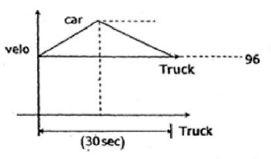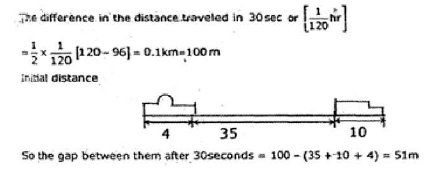## GATE Mechanical (ME) 2023 Mock Test Series

27 docs|243 tests
 Use Code STAYHOME200 and get INR 200 additional OFF Use Coupon Code